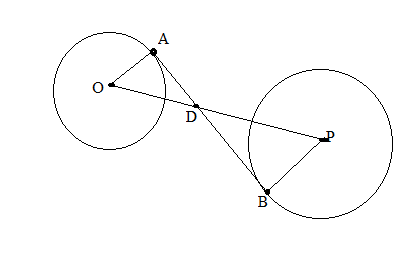Chapter 6.3, Problem 38EElementary Geometry For College St...

7th Edition
Alexander + 2 others
ISBN: 9781337614085

Solutions

Chapter
SectionElementary Geometry For College St...

7th Edition
Alexander + 2 others
ISBN: 9781337614085
Textbook Problem

The center of a circle of radius 3 in. is at a distance of 20 in. from the center of a circle of radius 9 in. what is the exact length of common internal tangent A B ¯ ?(HINT: Use similar triangles to find OD and DP. Then apply the Pythagorean Theorem twice.)Exercises 38, 39

To determine

To find:

The find value of AB¯.

Explanation

Given that, the center of a circle of radius 3 in. is at a distance of 20 in. from the center of a circle of radius 9 in.

That is OA=3,PB=9andOP=20 then AB¯ is common internal tangent.

The diagrammatic representation is given below,

Since AB¯ is common internal tangent therefore, AB¯OA¯andAB¯PB¯

Using the vertical angle theorem to get the following,

If two triangles are similar, then the ration of any two corresponding segments (such as altitudes, medians, or angle bisectors) equals the ratio of any two corresponding sides.

OA¯PB¯=OD¯PD¯39=OD¯PD¯OD¯PD¯=133OD¯=PD¯

We know that,

OP¯=OD¯+PD¯20=OD¯+PD¯PD¯=20OD¯. Since OP¯=20

Substitute this in the above equation to get the following,

Still sussing out bartleby?

Check out a sample textbook solution.

See a sample solution

The Solution to Your Study Problems

Bartleby provides explanations to thousands of textbook problems written by our experts, many with advanced degrees!

Get Started

let f(x) = x3 + 5, g(x) = x2 2, and h(x)= 2x + 4. Find the rule for each function. 2. f g

Applied Calculus for the Managerial, Life, and Social Sciences: A Brief Approach

Expand each expression in Exercises 122. (2xy)y

Finite Mathematics and Applied Calculus (MindTap Course List)

True or False: f(t) is used to measure the average rate of change of f with respect to t.

Study Guide for Stewart's Single Variable Calculus: Early Transcendentals, 8th